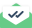# Zero angular velocities

Thank Dear Axel for your quick respond.

The older and newer versions are lammps-64bit-20160720 and
lammps-64bit-20160902 respectively.

I solved the problem by calculating angular velocities by adding the
following lines to the input file (only the z-component is shown here):

compute COM_Cu Cu com

variable dx atom x-c_COM_Cu

variable dy atom y-c_COM_Cu

variable dz atom z-c_COM_Cu

variable Lz atom (-vx*v_dy+vy*v_dx) # Angluar
momentum in z direction

compute Lz Cu reduce sum v_Lz

variable Iz atom (v_dx^2+v_dy^2) # Moment of

compute Iz Cu reduce sum v_Iz

variable W23 equal c_Lz/c_Iz # angular
velocity of Cu around z-axes

variable wz_NP equal omega(Cu,z) # angular
velocity of Cu around z-axes (by omega function)

compute w_NP Cu omega/chunk CHUNK # angular velocity of Cu
around z-axes (by omega/chunk compute)

The results show complete agreement between the results of the added lines
and both "compute omega/chunk" and "function omega()" which both show
exactly “zero” in the newer version. As you can see the angular velocities
are small but are not "zero":

step

c_w_NP

v_wz_NP

v_W23

0

1.46E-06

1.46E-06

1.46E-06

100

2.44E-06

2.44E-06

2.44E-06

200

7.17E-07

7.17E-07

7.15E-07

My original input file is very big so I send a small one to demonstrate the
problem.

Unfortunately, when my simulation box gets higher than a certain value, all
older versions of LAMMPS crash and show this message: "Imp-mpi.exe has
stopped working"

Fortunately, the newer version has not this problem.

Best regards

Dear all

The previous solution has a big problem: xyz should be in “unwrapped” form which are not accessible in “variable command”. This could lead to high level of error when a group of atoms is passing through a periodic boundary.

A simple new solution is explained below which uses unwrapped xyz: (of course, there would be no need to that when the problem of LAMMPS is solved by its developers)

By definition Angular momentum around z direction (Lz) is equal to inertia around z (Iz) times angular velocity in z direction (Wz):

Lz=Iz*Wz => Wz=Lz/Iz

The following lines do this for us:

compute AngMom all angmom/chunk CHUNK

compute Inertia all inertia/chunk CHUNK

variable W23 equal c_AngMom/c_Inertia

As shown below the results of these commands are in good agreement with “compute omega/chunk”:

step c_w_NP v_W23

0 1.46E-06 1.46E-06

100 2.44E-06 2.44E-06

200 7.17E-07 7.15E-07

300 2.03E-06 2.03E-06

Regards

MNSent with Mailtrack